## g A has all the mass at the rim, while wheel B has the mass uniformly distributed, like a solid disk. The wheels have the same mass. The sam

Question

g A has all the mass at the rim, while wheel B has the mass uniformly distributed, like a solid disk. The wheels have the same mass. The same torque is applied to both wheels. Which one accelerates faster in response to this torque?

in progress 0
6 months 2021-07-20T20:28:29+00:00 2 Answers 2 views 0

The rim accelerates faster than the disk in response to the torque.

Explanation:

Given:

For the rim:

mass = M

The moment of inertia is: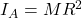For the disc:

mass = M

The moment of inertia is: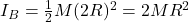If the same torque is applied, thus: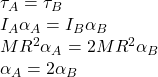According to this result, the rim accelerates faster than the disk.

The second wheel

Explanation:

The torque is given by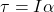(1)

where I is the moment of inertia and a is the angular acceleration. If we take into account the moment of inertia of a disk and a ring ()for the first wheel) we have: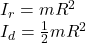where we used that both wheel have the same mass. By replacing in (1) we obtain: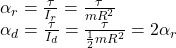Hence, the second wheel (the disk) has a greater acceleration.

hope this help!!